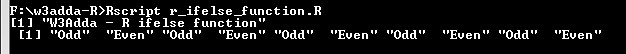# R ifelse() Function

In this tutorial you will learn about the R ifelse() Function and its application with practical example.

## R ifelse() Function

In R, the ifelse function is a vectorized version of standard R if..else statement. The ifelse function returns a value in the same shape as of the test expression. This vectorization makes it much faster than applying the same function to each of the vector element individually.

Syntax:-

expression :- Boolean Vector

x :- Return values for true elements of expression

y :- Return values for false elements of expression

Here, expression is a boolean vector (or coerced to boolean) and return value x and y is also vector with the same length as expression. The element `i` is x`[i]` if expression`[i]` is true, or y`[i]` if expression`[i]` is false.

Example:-

Output:-r_ifelse_function

In this tutorial we have learn about the R ifelse() Function and its application with practical example. I hope you will like this tutorial.